Courses

# Worksheet - Rational Numbers Class 7 Notes | EduRev

## Mathematics (Maths) Class 7

Created by: Praveen Kumar

## Class 7 : Worksheet - Rational Numbers Class 7 Notes | EduRev

The document Worksheet - Rational Numbers Class 7 Notes | EduRev is a part of the Class 7 Course Mathematics (Maths) Class 7.
All you need of Class 7 at this link: Class 7

Q.1. Find the Sum: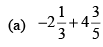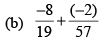Ans.

(a)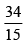(b)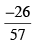Q.2. Find :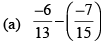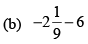Ans.

(a)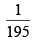(b)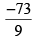Q.3. Find the product :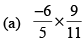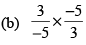Ans.

(a)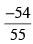(b) 1

Q.4. Find the value of :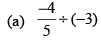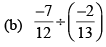Ans.

(a)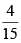(b)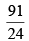Q.5. Fill int he boxes with the correct symbol out of >, < and =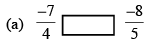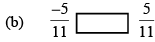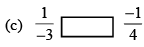Ans.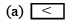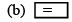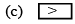Q.6. Which is greater in the following numbers?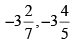Ans.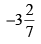Q.7. The product of two rational numbers is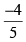If one of them is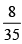find the other..

Ans.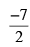Q.8. (a) What should be added to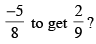(b) Find three rational numbers between 0 and 1.

Ans.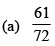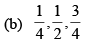Q.9. Find three rational numbers is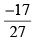If one fo them is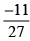find the other..

Ans.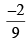10. Simplify :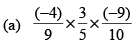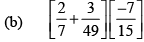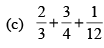(d) (4.3 – 2.3) × 6.3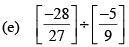Ans.

(a)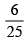(b)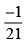(c)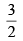(d)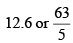(e)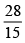Q.11. Arrange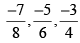in the ascending order..

Ans.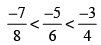Q.12. Find the additive inverse of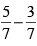Ans.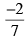Q.13. Find the multiplicative inverse of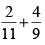Ans.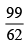Q.14. Find the reciprocal of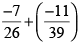Ans.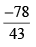Q.15. Jaspal donates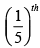part of his monthly income and deposited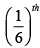part in the bank and expenditure the remaining income.
(a) Find the part of expenditure of his montly income.
(b) State two good habits of Jaspal mentioned on the basis of above questions.

Ans.

(a)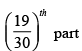(b) Two good habits : (1) Saving (2) Donation

Q.16. In the given figure represent the shaded region in the form of rational number.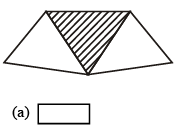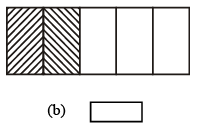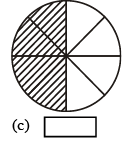Ans.

(a)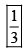(b)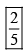(c)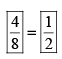,

,

,

,

,

,

,

,

,

,

,

,

,

,

,

,

,

,

,

,

,

;